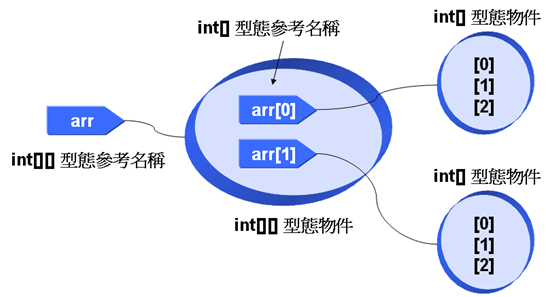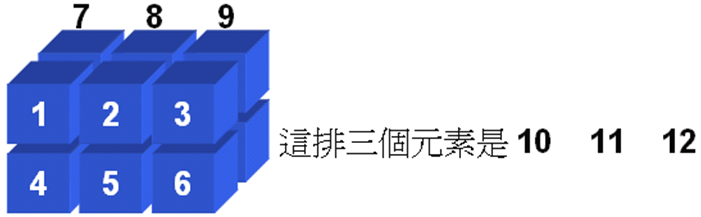# Java SE 6 技術手冊

## 5.1 一維陣列、二維陣列

### 5.1.1 一維陣列物件

``````int[] score = {90, 85, 55, 94, 77};
``````

#### 範例 5.1 SimpleArray.java

``````public class SimpleArray {
public static void main(String[] args) {
int[] score = {90, 85, 55, 94, 77};

for(int i = 0; i < score.length; i++)
System.out.printf("score[%d] = %d\n", i, score[i]);
}
}
``````

``````score = 90
score = 85
score = 55
score = 94
score = 77
``````

``````int[] arr = new int;
``````

byte 0
short 0
int 0
long 0L
float 0.0f
double 0.0d
char \u0000
boolean false

#### 範例 5.2 ArrayDemo.java

``````public class ArrayDemo {
public static void main(String[] args) {
int[] arr = new int;

System.out.print("arr 初始值: ");
for(int i = 0; i < arr.length; i++) {
System.out.print(arr[i] + " ");
arr[i] = i;
}

System.out.print("\narr 設定值: ");
for(int i = 0; i < arr.length; i++)
System.out.print(arr[i] + " ");
System.out.println();
}
}
``````

``````arr 初始值: 0 0 0 0 0 0 0 0 0 0
arr 設定值: 0 1 2 3 4 5 6 7 8 9
``````

``````int[] score = new int[] {90, 85, 55, 94, 77};
``````

#### 範例 5.3 SimpleArray2.java

``````public class SimpleArray2 {
public static void main(String[] args) {
int[] score = new int[] {90, 85, 55, 94, 77};

for(int i = 0; i < score.length; i++)
System.out.printf("score[%d] = %d\n", i, score[i]);
}
}
``````

#### 範例 5.4 AverageInput.java

``````import java.util.Scanner;

public class AverageInput {
public static void main(String[] args) {
Scanner scanner = new Scanner(System.in);

System.out.print("請輸入學生人數: ");

int length = scanner.nextInt();
float[] score = new float[length];  // 動態配置長度

for(int i = 0; i < score.length; i++) {
System.out.print("輸入分數：");
float input = scanner.nextFloat();
score[i] = input;
}

System.out.print("\n分數：");
float total = 0;
for(int i = 0; i < score.length; i++) {
total = total + score[i];
System.out.print(score[i] + " ");
}

System.out.printf("\n平均：%.2f", total / score.length);
}
}
``````

``````請輸入學生人數: 3

``````

### 5.1.2 二維陣列物件

``````int[][] arr = {{1, 2, 3},
{4, 5, 6}};
``````

#### 範例 5.5 TwoDimArray.java

``````public class TwoDimArray {
public static void main(String[] args) {
int[][] arr =     {{1, 2, 3},
{4, 5, 6}};

for(int i = 0; i < arr.length; i++) {
for(int j = 0; j < arr.length; j++)
System.out.print(arr[i][j] + " ");
System.out.println();
}
}
}
``````

``````1 2 3
4 5 6
``````

``````int[][] arr = new int;
``````#### 範例 5.6 TwoDimArray2.java

``````public class TwoDimArray2 {
public static void main(String[] args) {
int[][] arr = {{1, 2, 3},
{4, 5, 6}};

int[] foo = arr; // 將arr 所參考的陣列物件指定給foo

for(int i = 0; i < foo.length; i++) {
System.out.print(foo[i] + " ");
}
System.out.println();

foo = arr; // 將arr 所參考的陣列物件指定給foo

for(int i = 0; i < foo.length; i++) {
System.out.print(foo[i] + " ");
}
System.out.println();
}
}
``````

``````int[][] arr = new int[][] {{1, 2, 3},
{4, 5, 6}};
``````

``````int[][][] arr = {
{{1, 2, 3}, {4, 5, 6}},
{{7, 8, 9}, {10, 11, 12}}
};
````````````int[][][] arr = new int;
``````

#### 範例 5.7 TwoDimArray3.java

``````public class TwoDimArray3 {
public static void main(String[] args) {
int arr[][];

arr = new int[];
arr = new int; // arr 參考至長度為3的一維陣列
arr = new int; // arr 參考至長度為5的一維陣列

for(int i = 0; i < arr.length; i++) {
for(int j = 0; j < arr[i].length; j++)
arr[i][j] = j + 1;
}

for(int i = 0; i < arr.length; i++) {
for(int j = 0; j < arr[i].length; j++)
System.out.print(arr[i][j] + " ");
System.out.println();
}
}
}
``````

``````1 2 3
1 2 3 4 5
``````